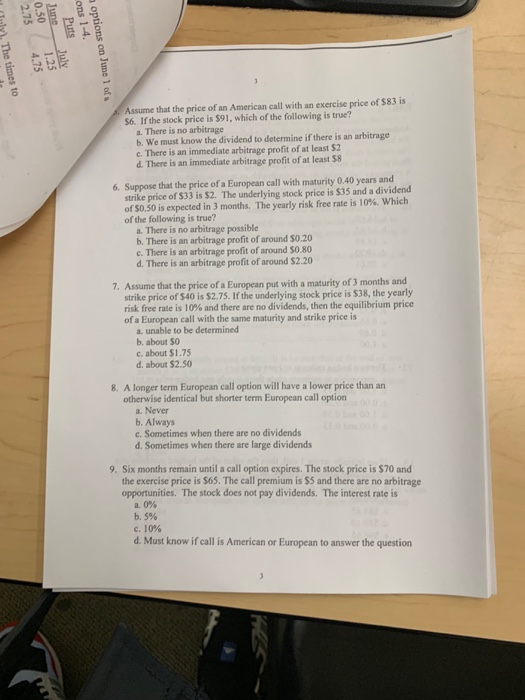### Create an Account

Home / Questions / 2 75 0 50 June Puts ons 1-4 Inly The times to 475 125 options on June 1 of a Assume that ...

# 2 75 0 50 June Puts ons 1-4 Inly The times to 475 125 options on June 1 of a Assume that the price of an American call2.75 0.50 June Puts ons 1-4. Inly. The times to 4.75 1.25 options on June 1 of a Assume that the price of an American call with an exercise price of 583 is 56. If the stock price is \$91, which of the following is true? a. There is no arbitrage b. We must know the dividend to determine if there is an arbitrage c. There is an immediate arbitrage profit of at least 52 d. There is an immediate arbitrage profit of at least 58 6. Suppose that the price of a European call with maturity 0.40 years and strike price of 533 is \$2. The underlying stock price is \$35 and a dividend of \$0.50 is expected in 3 months. The yearly risk free rate is 10%. Which of the following is true? a. There is no arbitrage possible b. There is an arbitrage profit of around \$0.20 c. There is an arbitrage profit of around 50.80 d. There is an arbitrage profit of around \$2.20 7. Assume that the price of a European put with a maturity of 3 months and strike price of \$40 is \$2.75. If the underlying stock price is \$38, the yearly risk free rate is 10% and there are no dividends, then the equilibrium price of a European call with the same maturity and strike price is a. unable to be determined b, about 50 c. about \$1.75 d. about \$2.50 8. A longer term European call option will have a lower price than an otherwise identical but shorter term European call option a. Never b. Always c. Sometimes when there are no dividends d. Sometimes when there are large dividends 9. Six months remain until a call option expires. The stock price is \$70 and the exercise price is \$65. The call premium is \$5 and there are no arbitrage opportunities. The stock does not pay dividends. The interest rate is a. 0% b. 5% c. 10% d. Must know if call is American or European to answer the question

Feb 08 2020 View more View LessGet Solution